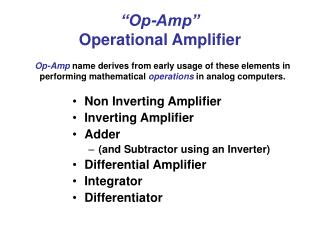DownloadDownload Presentation“Op-Amp” Operational Amplifier

# “Op-Amp” Operational Amplifier

Download Presentation## “Op-Amp” Operational Amplifier

- - - - - - - - - - - - - - - - - - - - - - - - - - - E N D - - - - - - - - - - - - - - - - - - - - - - - - - - -
##### Presentation Transcript

1. “Op-Amp”Operational Amplifier • Non Inverting Amplifier • Inverting Amplifier • Adder • (and Subtractor using an Inverter) • Differential Amplifier • Integrator • Differentiator Op-Amp name derives from early usage of these elements in performing mathematical operations in analog computers.

2. Three Ways to Examine Op-Amp Behavior • Consider as an Ideal Op-Amp Component • Consider as a Feedback Model and Examine Behavior • Perform Conventional Circuit Analysis

3. VE = VIN+ - VIN- VOUT = a * VE VIN- VIN+

4. Ideal Op-Amp Model VE = VIN+ - VIN- VOUT = a * VE

5. Behavior of Feedback Model

6. Behavior of Feedback Model of Non Inverting Amplifier

7. Behavior of Feedback Model

8. Behavior of Feedback Model

9. Behavior of Feedback Model

10. Behavior of Feedback Model Summary

11. Circuit Analysis Approach

12. Circuit Analysis Approach

13. “Op-Amp”Operational Amplifier • Non Inverting Amplifier • Inverting Amplifier • Adder • (and Subtractor using an Inverter) • Differential Amplifier • Integrator • Differentiator Op-Amp name derives from early usage of these elements in performing mathematical operations in analog computers.

14. a (V+ - V-) Differential Amplifier Circuit Analysis

15. a (V+ - V-) Differential Amplifier Circuit Analysis

16. a (V+ - V-) Differential Amplifier Circuit Analysis

17. a (V+ - V-) Differential Amplifier Circuit Analysis

18. a (V+ - V-) Differential Amplifier Circuit Analysis  ZF/ ZG

19. v1 v1 vid / 2 v2  vid / 2 vicm v2 Model of inputs with common-mode and differential-mode components Original Inputs vi1 vi2 Common Mode Rejection Ratio

20. Common Mode Rejection RatioCMRR whereAis the differential mode gain andAcmis the common mode gain Ideally:CMRR Typically: 60 dB  CMRR  120 dB

21. Assumes R2 = R4 and R1 = R3

22. Differential Amplifier Circuit Analysis with Component Imbalance

23. Differential Amplifier Circuit Analysis with Component Imbalance

24. Differential Amplifier Circuit Analysis with Component Imbalance

25. Differential Amplifier Circuit Analysis with Component Imbalance

26. Differential Amplifier Circuit Analysis with Component Imbalance

27. The Maximum Power Transfer Theorem simply states, the maximum amount of power will be dissipated by a load resistance when that load resistance is equal to the Thevenin/Norton resistance of the network supplying the power.

28. To create the Thevenin Equivalent Circuit we need: • Value of the Thevenin Voltage Source • Value of the Thevenin Resistance

29. - Ro vo Rd vd ii + io Avd - vi RL + CL Input and Output Impedances of Noninverting Op-amp Configuration The unity gain buffer input impedance is much higher than the op-amp input impedance Rd. The amplifier output impedance is much smaller than the op-amp output impedance Ro.

30. R2 R2 vref R3 R3 R4 R4 v1 v2 vout R1 Instrumentation Amplifier

31. Instrumentation Amplifier Example Burr-Brown INA118 Parameters: Gain:

32. R2 R2 C R3 R3 R4 R4 R v1 v2 vout 2R1 Instrumentation Amp (cont.) A feedback network may also be included with the instrumentation amplifier. vdiff = v2 - v1